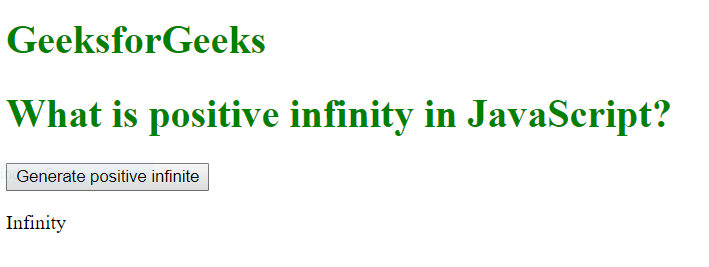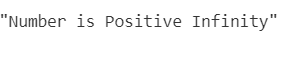# What is positive infinity in JavaScript?

The positive infinity in Javascript is a number which is constant and represents a value which is highest available.It can be generated using a self made function or by an arithmetic operation.

Note: JavaScript shows the POSITIVE_INFINITY value as Infinity.

Positive Infinity is different from mathematical infinity in the following ways:

1. The product of two positive infinity is positive infinity
2. Product of positive and negative infinity is negative infinity
3. If we divide any positive number by positive infinity, we will get positive 0
4. If we divide any negative number by positive infinity, we will get negative 0
5. 0 multiplied by positive infinity is NaN
6. NaN multiplied by positive infinity is NaN
7. We get negative infinity when we divide positive infinity by any negative number (except negative infinity)
8. We get positive infinity when we divide positive infinity by any positive number (except positive infinity)
9. Positive infinity divided by either positive or negative infinity is NaN

Syntax:

`Number.POSITIVE_INFINITY`

Example 1:

 ` ` `<``html``> ` ` `  `<``body``> ` `    ``<``style``> ` `        ``h1 { ` `            ``color: green; ` `        ``} ` `    `` ` ` `  `    ``<``h1``>GeeksforGeeks ` `    ``<``h1``>What is positive infinity in JavaScript? ` ` `  `    ``<``button` `onclick``=``"geekPositiveInfinity()"``> ` `      ``Generate positive infinite ` `  `` ` ` `  `    ``<``p` `id``=``"geek"``> ` ` `  `    ``<``script``> ` `        ``function geekPositiveInfinity() { ` `           `  `           ``//positive value greater than the largest ` `           ``// representable number in JavaScript ` `            ``var n = (Number.MAX_VALUE) * 2; ` `            ``document.getElementById("geek").innerHTML = n; ` `        ``} ` `    `` ` ` `  ` ` ` `  ` `

Output:Example 2:

 ` `

Output:Supported Browsers:

• Mozilla Firefox
• Apple Safari
• Opera
• Internet Explorer/EdgeMy Personal Notes arrow_drop_upCheck out this Author's contributed articles.

If you like GeeksforGeeks and would like to contribute, you can also write an article using contribute.geeksforgeeks.org or mail your article to contribute@geeksforgeeks.org. See your article appearing on the GeeksforGeeks main page and help other Geeks.

Please Improve this article if you find anything incorrect by clicking on the "Improve Article" button below.